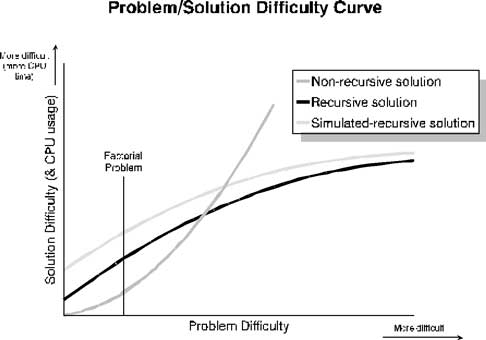Problem solve Get help with specific problems with your technologies, process and projects.

# Solving difficult problems with recursion -- Part II

## In Part I of this series, Ron Turull discussed what recursion is and how it works. Now he looks at how to implement it programmatically.Ron TurullIn Part I of this series, we discussed what recursion is and how it works. Now let's look at how to implement it programmatically.

How to code a recursive solution
The easiest and most efficient way to code a recursive solution is through recursive program calls. (A recursive call is when a program calls itself either directly or indirectly.) As mentioned, though, ILE RPG does not currently support recursive program calls, but it does support recursive procedure calls. This works great for the factorial problem, since it does not involve files (more on files later).

The code, fact_rec in ront/qrpglesrc, is an RPG procedure that illustrates a recursive solution to the factorial problem. The basic formula for coding a recursive solution is as follows:

0. Check for error condition. This may or may not be necessary depending on the problem. It was necessary for the factorial problem because the solution to the factorial of a non-positive integer is undefined.

1. If the problem is a trivial case, continue with step 2, else goto step 3.

2. Solve trivial case problem. Go to 4.

3. Divide problem into one or more subproblems. Call same program/procedure for each subproblem. Combine the result(s) returned by the called program/procedure.

4. Return solution to caller or use internally (whatever the case may be).

You can compare this to the RPG do-loop implementation shown here in fact_do in ront/qrpglesrc. Because the factorial problem is a simple one, the do-loop implementation is just as simple as the recursive one just discussed. It runs more efficiently than the recursive procedure, since it doesn't contain any procedure calls. However, that is not generally the case. As the difficulty of the problem (and its solution) increases, a recursive solution (if there is one) becomes more efficient than a non-recursive solution. That leads us to the principle of practicality.The principle of practicality
As the factorial example illustrated, not all problems that have a recursive solution should necessarily be solved recursively. In fact, many problems can have both a recursive and a non-recursive solution. When deciding which solution to use, you must keep in mind not only the degree of difficulty of the resulting algorithm, but also the programming code necessary to implement it.

You also must consider if the solution involves files, specifically whether or not you need to have a new file pointer for each level of the recursion. If so, you will either have to use a language that supports true recursion, such as C, or you will have to simulate recursion in RPG, which will add another layer of difficulty to the solution. This then constitutes the principle of practicality and is usually a function of the difficulty of the problem itself.

The figure below graphically illustrates this principle. It applies only to problems that can be solved recursively. As the problem becomes more difficult, the difficulty and CPU usage of the non-recursive solution begin to soar. On the other hand, the difficulty and CPU usage of the recursive solution -- simulated or otherwise -- begins to flatten. Notice that for very simple problems, the non-recursive approach is usually better.The graph also illustrates that simulated recursion is more costly than true recursion. This extra cost is due to the extra effort necessary to code and maintain a simulated recursive program, not to greater CPU usage. (In fact, in many instances CPU usage may be less with the simulated approach because there will not be any recursive program calls.) Regardless, the two lines start to converge as the cost difference becomes negligible.

Three important things to remember about recursion
Keep in mind these three important principles:

1. Implementing a non-recursive solution to a difficult problem (one that has a recursive solution) can overly tax your human resources as well as your machine resources.
2. Except for the simplest problems, a true recursive solution simplifies code.
3. Simulated recursive solutions require more complicated coding, requiring a greater degree of problem difficulty before they become practical.

A problem to ponder
Want another example? Think about how you might find the Nth number in the Fibonacci series, both recursively and non-recursively. The formula for the Fibonacci series is FIB(N) = FIB(N-1) + FIB(N-2). That is, the Nth number in the Fibonacci series is simply the sum of the two immediately preceding numbers in the series. FIB(2) and FIB(1) are the trivial cases, and both are equal to 1. (Yes, there can be more than one trivial case.) So, FIB(3) = FIB(2) + FIB(1) = 1 + 1 = 2, FIB(4) = 3, FIB(5) = 5, FIB(6) = 8, and so on.

-----------------------------------
About the author: Ron Turull is editor of Inside Version 5. He has more than 20 years' experience programming for and managing AS/400-iSeries systems.

#### Start the conversation

Send me notifications when other members comment.

## SearchDataCenter

• ### Advances in server hardware components speed up data centers

Server hardware has consistently evolved since the 1980s. Now, IT professionals can implement the latest in CPU and GPU ...

• ### Improvements in CPU features help shape selection

CPUs have evolved to meet ever-increasing technology demands. We look at the way performance and power characteristics have ...

• ### Dive into the quantum computing qubit with IBM's Bob Sutor

The quantum computing industry is entering a new era. IBM's Bob Sutor discusses the technology's importance and how his latest ...

Close